# Quiz 6: Human Capital

Business

a) First, Debbie will compare the present value of income for each career option and choose the one that has the highest present value. If the interest rate is 5 percent,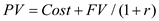Where PV = Present Value of each career choice Cost = Amount of cost incurred FV = Future value r = Rate of interest Substituting the above values in the formula, we get;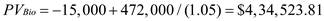and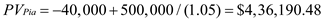From the above results, we can see that the present value of concert pianist (\$ 4, 36,190.48) is greater than that of being a biologist (\$ 4, 34,523.81). Therefore, she will become a concert pianist. If the rate of interest is 15 percent, however, the present value calculations become;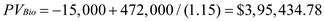and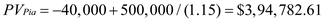In this case, Debbie will choose to become a biologist because the present value of being a biologist (\$ 3, 95,434.78) is higher than a pianist (\$ 3, 94,782.61). As the interest rate increases, the worker discounts future earnings more, lowering the returns from investing in education. b) Debbie will compare the present value of being a biologist from part (a) with the present value of becoming a pianist. The relevant present values are: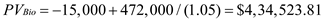and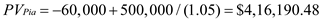Debbie will, therefore, become a biologist, showing that as the cost of an invest increases, the chance of pursuing that investment falls. This concludes your solution.

The notion of present value helps us to compare the dollar amounts spend and received in different time periods. This is because the value of a dollar received today is not equivalent to the value of dollar received tomorrow. The formula for calculating the present value is as follows: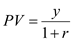Where PV = Present Value y = Future value r = Rate of interest or rate of discount In this formula, the quantity PV tells us how much need to be invested today in order to have y dollars next year. However, a future payment of y dollars is discounted so as it makes it comparable to current dollars. This concludes your solution.

The present discounted values of Peter's earnings associated with each of the alternatives are;Where PV = Present Value of each career choice Cost = Amount of cost incurred FV = Future value r = Rate of interest Substituting the above values in the formula, we get;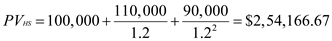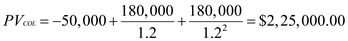and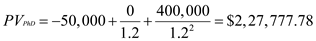Thus, the best option for Peter is to start working upon completely high school. This is because the present value for working immediately is the greatest (\$ 2, 54,166.67) amongst all the three alternatives. This concludes your solution.